# Algorithms for computing sums of powers of arithmetic progressions by using Eulerian numbers

Peter J. Shiue, Shen C. Huang and Jorge E. Reyes
Notes on Number Theory and Discrete Mathematics
Print ISSN 1310–5132, Online ISSN 2367–8275
Volume 27, 2021, Number 4, Pages 140—148
DOI: 10.7546/nntdm.2021.27.4.140-148

## Details

### Authors and affiliations

Peter J. ShiueDepartment of Mathematical Sciences, University of Nevada, Las Vegas
4505 S. Maryland Pkwy. Las Vegas, NV, 89154, United States of America

Shen C. HuangDepartment of Mathematical Sciences, University of Nevada, Las Vegas
4505 S. Maryland Pkwy. Las Vegas, NV, 89154, United States of America

Jorge E. ReyesDepartment of Mathematical Sciences, University of Nevada, Las Vegas
4505 S. Maryland Pkwy. Las Vegas, NV, 89154, United States of America

### Abstract

The sums of powers of arithmetic progressions is of the form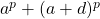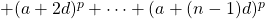, where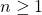,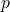is a non-negative integer, and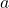and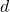are complex numbers with. This sum can be computed using classical Eulerian numbers  and general Eulerian numbers . This paper gives a new method using classical Eulerian numbers to compute this sum. The existing formula that uses general Eulerian numbers are more algorithmically complex due to more numbers to compute. This paper presents and focuses on two novel algorithms involving both types of Eulerian numbers. This paper gives a comparison to Xiong et al.‘s result with general Eulerian numbers . Moreover, an analysis of theoretical time complexities is presented to show our algorithm is less complex. Various values ofare analyzed in the proposed algorithms to add significance to the results. The experimental results show the proposed algorithm remains around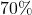faster asincreases.

### Keywords

• Analysis of algorithms
• Sums of powers of arithmetic progressions
• Dynamic programming
• Classical Eulerian numbers
• General Eulerian numbers

• 05A10
• 11B25
• 11B65
• 68N15
• 68Q25

### References

1. Bounebirat, F., Laissaoui, D., & Rahmani, M. (2018). Several explicit formulae of sums and hyper-sums of powers of integers. Online Journal of Analytic Combinatorics, 13(4), Article 4 (9 pages).
2. Carlitz, L. (1959). Eulerian numbers and polynomials. Mathematics Magazine, 32(5), 247–260.
3. Charalambides, C. A. (2002). Enumerative Combinatorics. Chapman & Hall / CRC Press.
4. Comtet, L. (1974). Advanced Combinatorics: The Art of Finite and Infinite Expansions. Springer Science & Business Media.
5. Hsu, L. C., & Shiue, P. J. (1999). On certain summation problems and generalizations of Eulerian polynomials and numbers. Discrete Mathematics, 204(1–3), 237–247.
6. Laissaoui, D., & Rahmani, M. (2017). An explicit formula for sums of powers of integers in terms of Stirling numbers. Journal of Integer Sequences, 20(4), Article 17.4.8 (6 pages).
7. Lehmer, D. (1982). Generalized Eulerian numbers. Journal of Combinatorial Theory, Series A, 32(2), 195–215.
8. Pita-Ruiz, C. (2018). On a generalization of Eulerian numbers. Notes on Number Theory and Discrete Mathematics, 24(1), 16–42.
9. Shiue, P. J., Huang, S. C., & Jameson, E. (2020). On algorithms for computing the sums of powers of arithmetic progressions. Notes on Number Theory and Discrete Mathematics, 26(4), 113–121.
10. Vassilev, P., & Vassilev-Missana, M. (2005). On the sum of equal powers of the first n terms of an arbitrary arithmetic progression. Notes on Number Theory and Discrete Mathematics, 11(3), 15–21.
11. Worpitzky, J. (1883). Studien uber die Bernoullischen und Eulerschen Zahlen.  Journal fur die reine und angewandte Mathematik, 94, 203–232.
12. Xiong, T., Tsao, H.-P., & Hall, J. I. (2013). General Eulerian numbers and Eulerian polynomials. Journal of Mathematics, 2013, 1–9.

## Cite this paper

Shiue, P. J., Huang, S. C., & Reyes, J. E. (2021). Algorithms for computing sums of powers of arithmetic progressions by using Eulerian numbers. Notes on Number Theory and Discrete Mathematics, 27(4), 140-148, doi: 10.7546/nntdm.2021.27.4.140-148.